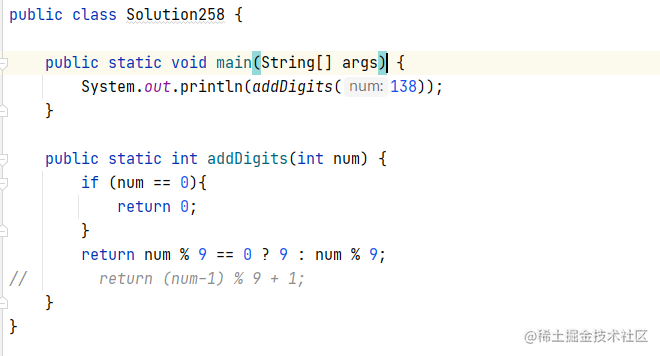# 上机题 leetcode 题目 给定一个非负整数 num，反复将各个位上的数字相加，直到结果为一位数 并且时间和空间复杂度都为o(1)

## 示例

``````输入: 38

• 若a+b+c < 9，则a+b+c =  (a+b+c)%9 = abc%9 即为结果；
• 若a+b+c > 9，令de = a+b+c，de%9 = (d+e)%9：
• 若d+e > 9，令fg = d + e，fg%9 = (f+g)%9：
• .... ....若干步后，一定有【xy%9 = (x+y)%9 = 9】即为结果或【xy%9 = (x+y)%9 <9】
• 故当abc%9 = 0，结果为9;当abc%9 != 0时,则结果为abc%9。有更好的解法，欢迎评论指导